[BACK]Computer Modeling in Engineering & SciencesDOI: 10.32604/cmes.2022.017304

ARTICLE

Bioconvection Mangnetohydrodynamic Tangent Hyperbolic Nanofluid Flow with Quartic Chemical Reaction Past a Paraboloid Surface

1Department of Mathematics, Capital University of Science and Technology, Islamabad, Pakistan
2Department of Mechanical Engineering, College of Engineering, Prince Mohammad Bin Fahd University, Al Khobar, Saudi Arabia
3Department of Mathematics, University of Sargodha, Sargodha, Pakistan
4CAS Key Laboratory of Mechanical Behavior and Design of Materials, Department of Modern Mechanics, University of Science and Technology of China, Hefei, China
Received: 30 April 2021; Accepted: 07 June 2021

Abstract: In this numerical study, the effect of quartic autocatalysis type of chemical reaction, buoyancy force and thermal radiation phenomenon and magnetic effect on tangent hyperbolic nanofluid past an upper horizontal surface of a paraboloid has been studied. By considering the Buongiorno model approach, a diffusion of unequal coefficients in the presence of gyrotactic microorganism is discussed. Implementation of microorganism's idea is used to stabilize the nanoparticles through bioconvection. The modeled PDEs of the problems are converted into nonlinear ODEs with the assistant of the similarity transformations. To tackle nonlinear ODEs, MATLAB package bvp4c is used. In addition, a hallmark of the Matlab code with the reported results in the literature is achieved by benchmarking. The variations in motion, concentration, temperature, and motile density due to sundry parameters have been analyzed in-depth via graphs. Our analysis shows that the density profile of motile of microorganism is hiked with an increment in the bioconvection Rayleigh number but decreases for higher thermal Grashof number.

Keywords: Quartic autocatalysis; tangent hyperbolic nanofluid; brownian motion; paraboloid of revolution; MHD flow; thermophoresis

1  Introduction

The magnetohydrodynamic (MHD) studies have drawn substantial interest from researchers and scientists due to their vast applications in engineering and industry. The use of MHD in steaming, melting, stirring, and levitating liquid metals is very frequent. MHD has recently been shown to be helpful in many disease diagnosis processes. Flows under the influence of MHD, therefore attract significant interest from many researchers. As a consequence, MHD flows attain a great deal of focus from many researchers. A few of the recent developments are as follows: Hassan et al.  performed the comparison of non-magnetic and magnetic effect on nanoparticles past a wedge. Slip and thermal radiation effects on the MHD Casson effect were investigated by Raza  with concluding remarks that the concentration boundary layer thickness is hiked as the chemical reaction parameter gets higher. Yashkun et al.  highlighted the MHD hybrid radiative nanofluid past a shrinking/stretching surface. One of the conclusions was that suction parameter enhances the shrinking/stretching parameter range for which the solution exists. Anuar et al.  performed the stability analysis of magnetohydrodynamics flow past a deforming vertical surface and reported that only the first solution is linearly stable. Further detail in this regard can be seen in .

Bioconvection is the development of random patterns due to the swimming of the microorganisms. This bioconvection phenomenon plays a vital role in the industry, food digestion, agriculture and antibiotics. Their inclusion in the base fluids improves the transfer of mass significantly. Kuznetsov et al. [21,22] presented the natural convection flow of nanofluid past a vertical sheet. Nima et al.  reported the behavior of non-Newtonian fluid with variable properties in the presence of the gyrotactic microorganisms. One of the key features was that the density profile is upsurged as the bioconvection Lewis number gets higher. Khan et al.  reported the Jaffery nanofluid with gyrotactic microorganisms in the presence of effective Prandtl number and Activation energy. The impact of heat generation on bioconvection MHD nanofluid flow was analyzed by Kotha et al. . Atif et al.  examined the MHD micropolar fluid with stratification effects in the presence of nanoparticles and gyrotactic microorganisms with concluding remarks that density distribution is rambled as each of the mass stratification and buoyancy ratio parameter is boosted. When two or more reactants yield products, a chemical reaction occurs. This chemical reaction may be homogenous or heterogeneous depending upon whether the reaction takes place at the interface or due to a single-phase volume reaction . Lotka  presented the idea of an autocatalytic chemical reaction. In this perspective, the simplest one is X + Y → Y + Y, which means that species X interacts with specie Y, and in the final product, whole species X is converted into species Y. Sapre  presented the idea of a quadratic and cubic autocatalytic chemical reaction. Animasaun et al.  analyzed the viscoelastic fluid flow by considering first-order reaction and cubic autocatalytic reaction schemes. The variable thickness of an object is one of the significant properties of the materials. In industry, there are many applications where the fluid flows over a non-uniform thickness surface. Patil et al.  looked in the double-diffusive flow past a stretching surface of variable thickness. One of the key findings of this study was that the enhancement in the Prandtl number depresses the 30% of the thermal boundary thickness. Qasim et al.  scrutinized the numerical and analytical solution of the mixed convection flow past a vertical sheet of variable thickness. One of the main observations of that study was that an increment in the surface drag is noticed as the wall thickness parameter is enhanced. Three-dimensional MHD Carreau fluid flow over a paraboloid surface with thermal radiation effects was inspected by Abdeljawad et al. . An upper paraboloid surface (ups) phenomenon of bioconvection in Carreau fluid was discussed by Khan et al.  and conclude that velocity profile is declined as the bioconvection Rayleigh number is escalated. For non-Newtonian fluids past a paraboloid surface of revolution, flow properties were discussed by Santoshi et al. .

In the above-presented literature, it is noticeable that numerous researchers investigated the different non-Newtonian fluid flows past different geometries. However, no one studied the tangent hyperbolic nanofluid flow over a paraboloid surface with gyrotactic microorganisms. In this numerical computation, a tangent hyperbolic nanofluid past a horizontal paraboloid surface with a quartic autocatalytic type of chemical reaction is analyzed. The arising non-linear ODEs are tackled with Matlab package bvp4c which is a finite difference code that implements the 3-stage Lobatto IIIa formula. To use bvp4c from Matlab, first, Eqs. (15)(19) are transformed into a set of a coupled first-order system of equations. This transformation is used to set up the system of equations as a boundary value problem (BVP) and use the BVP solver in Matlab to numerically solve this system, with the above boundary condition and assumed a suitable finite value for the far-field boundary condition, i.e., η, say η=25. In solving the present problem using bvp4c from Matlab, bvp4c has three arguments: a function odes for evaluating the ODEs, a function BCS for evaluating the residual in the boundary conditions, and a structure solicit that provides a guess for a mesh and the solution on this mesh. The ODEs are handled exactly as in the Matlab IVP solvers. Numerical results are obtained for the different values of the dimensionless governing parameters. Graphical representations of dimensionless quantities like velocity, concentration density profile, and temperature are investigated in detail.

2  Formulation of the Governing Equations

Tangent hyperbolic nanofluid flow past ups in the presence of the gyrotactic microorganisms are analyzed. The flow analysis is carried out in the presence of magnetic and thermal radiation effects. For the stability of the nanoparticles, microorganisms are induced. The layer very next to ups is parallel to the sheet having velocity u=Uw=U0(x+b)m. The start of the fluid flow is not from the origin. Therefore, y=A(x+b)1m2 is taken as the start of the flow where m<1 is the velocity index. The components of the velocities, temperature, and density of motile microorganisms are presented as u(x,y), v(x,y), T(x,y), and n(x,y), respectively. Two reactants X and Y, have concentrations C1 and C2, react with each other, and are such that the concentration of Y is higher than the cubic scheme, i.e., the concentration of the reactant X. A chemical change occurs in reactants X and Y at the interface, due to which homogenous the catalytic reaction is properly accounted for in the boundary layer. Considering the concept of homogeneous-heterogeneous reaction model [36,37], isothermal quartic autocatalysis type of chemical reaction inside the boundary layer for the higher concentration of reactant Y at the surface was proposed as X+3Y4Y with the rate of chemical reaction =kC1C23. In the presence of the catalyst at the paraboloid surface, a single isothermal first-order chemical reaction exists and is the form XY with the rate of chemical reaction =kC1. The domain of the tangent hyperbolic nanofluid flow is considered as (x+b)1m2y< as presented in Fig. 1. A magnetic field B=B0(x+b)m12 is applied and considering the idea of the Rosseland approximation, thermal radiation is also incorporated. We assume the temperature difference within the flow is small. Therefore, in this perspective, T4 in radiative heat flux is linearized about T and higher-order terms are neglected.Figure 1: Flow configuration

The governing equations of the above-modeled problem

ux+vy=0,(1)

uux+vuy=ν[(1n)+2nΓ(uy)]2uy2+x(gxωm+12(TT))+x(gxβ(ρmρ)m+12)(NN)σB2(x)ρu,(2)

uTx+vTy=α2Ty21ρCpqry+(ρCp)p(ρCp)f[DBC1yTy+DTT(Ty)2],(3)

uC1x+vC1y=D12Cy2DTT2Ty2K1C1C23,(4)

uC2x+vC2y=D22Cy2+DTT2Ty2+K1C1C23,(5)

uNx+vNy+by(NWcΔC1C1y)=Dm(2Ny2).(6)

The boundary conditions are as follows

u(x,y)=U0(x+b)m,    v(x,y)=0,    T(x,y)=Tw,  D1C1y=KsaC1,   D2C2y=KsaC1,    N(x,y)=Nw} at    y=A(x+b)1m2, u(x,y)0,    T(x,y)T,       C1C0,       C20,      NN       as     y}(7)

In order to obtain the nondimensional form of the modeled equations, a stream function ψ, similarity variable ζ, dimensionless temperature θ, dimensionless density motile of microorganism l, dimensionless concentration of reactant X and reactant Y is denoted by h, and g respectively are of the following form:

ζ=y(m+1)U02ν(x+b)m12,    ψ=2νU0(m+1)(x+b)m+12f(ζ),θ(ζ)=TTTwT,l(ζ)=NNNwN,    h(ζ)=C1C,    g(ζ)=C2C.}(8)

Here ζ denotes the dimensionless distance, ψ the stream function, θ(ζ) the dimensionless temperature, l(ζ) the dimensionless density of the motile microorganism, h(ζ) concentration of the homogeneous fluid and g(ζ) concentration of the fluid hetrogeneous.

It is noticeable that the continuity equation is satisfied automatically, and locally transformed ODEs are as follows:

(1n+nWed2fdζ2)d3fdζ32mm+1dfdζdfdζ+fd2fdζ2+Grθ+Rbl2m+1Mdfdζ=0,(9)

(1+43Rd)d2θdζ2+Pr[fdθdζ+(1n)Ecd2fdζ2d2fdζ2+n2EcWed2fdζ2d2fdζ2d2fdζ2+2m+1MEcdfdζdfdζ+Nbdθdζdhdζ+Ntdθdζdθdζ]=0,(10)

d2hdζ2+Sc1fdhdζNtNbd2θdζ22mm+1Sc1Khg3=0,(11)

δd2gdζ2+Sc2fdgdζ+NtNbd2θdζ2+2mm+1Sc2Khg3=0,(12)

d2ldζ2Sm1m1+mdfdζl+SmfdldζlPed2hdζ2Pedgdζdldζ=0,(13)

It is noticeable that the least value of y is not the starting point of the slot. It is considering this fact, the BCs (7) cannot be imposed at y=0. It is unrealistic to impose y=0 to the surface of variable thickness. Therefore y=0 is not applicable in variable ζ. By substitution y=A(x+b)1m2, as least value of y which corresponds to the smallest value of similarity variable χ is given by χ=AU0(m+1)2ν. Therefore, for scaling of BCs at wall we consider ζ=χ.

The BCs becomes:

f(χ)=1,f(χ)=χ1m1+m,θ(χ)=1,h(χ)=Λh,δg(χ)=Λh(χ),l(χ)=1atχ=ζ,f(χ)0,θ(χ)0,g(χ)0,h(χ)1l(χ)0asχ.}(14)

In the above equations, We=(m+1)U03(x+b)3m1ν the Weissenberg number, Gr=gω(TwT)U02(x+b)2m1 the Grashof number, M=σB02aρ is the magnetic number, Rd=4δT3kk the thermal radiation parameter, Pr=να the Prandtl number, Nb=(ρCp)pDB(CwC)(ρCp)fν the Brownian motion parameter, K=K1C0C02U0(x+b)m1 strength of the homogeneous reaction, Nt=(ρCp)pDT(TwT)(ρCp)fνT the thermophoresis parameter, Rb=gxβ(ρmρ))(NwN)U02(x+b)2m1 bioconvection Rayleigh number, Sc2=νD2 the Schmidt number related to reactant Y, Ec=U02(x+b)2m(Cp)f(TwT) the Eckert number, Λ=Ksa(m+12U0ν)12D1(x+b)m12 strength of the heterogeneous reaction, Nt=(ρCp)pDT(TwT)(ρCp)fνT the thermophoresis parameter, Sc1=νD1 the Schmidt number related to reactant X, Pe=bWcDm the bioconvection Peclet number, Sm=νDn the Schmidt number for motile microorganism and δ=D2D1 the ratio of diffusion coefficients of reactant X and Y.

The dimensionless modelled equation Eqs. (9)–(13) are depending on ζ whereas the BCs (14) are depending on χ. Therefore to transform the domain from [χ,) to [0,), it is valid to adopt F(ζ)=F(χζ)=f(η), Θ(ζ)=Θ(χζ)=θ(η), H(ζ)=H(χζ)=h(η), G(ζ)=G(χζ)=G(η) and L(ζ)=L(χζ)=l(η).

(1n+nWed2Fdη2)d3Fdη32mm+1dFdηdFdη+Fd2Fdη2+GrΘ+RbL2m+1MdFdη=0,(15)

(1+43Rd)d2Θdη2+Pr[FdΘdη+(1n)Ecd2Fdη2d2Fdη2+n2EcWed2Fdη2d2Fdη2d2Fdη2+2m+1MEcdFdηdFdη+NbdΘdηdHdη+NtdΘdηdΘdη]=0,(16)

d2Hdη2+Sc1FdHdηNtNbd2Θdη22mm+1Sc1KHG3=0,(17)

δd2Gdη2+Sc2FdGdη+NtNbd2Θdη2+2mm+1Sc2KHG3=0,(18)

d2Ldη2Sm1m1+mdFdηL+SmFdLdηLPed2Hdη2PedHdηdLdη=0,(19)

subject to BCs

dFdη=1,F(η)=χ1m1+m,Θ(η)=1,dHdη=ΛH,δdGdη=ΛH(η),L(η)=1,}atη=0,dFdη0,Θ(η)0,H(η)1,G(η)0,L(η)0asη.}(20)

3  Engineering Quantities

In engineering and industry, the quantities which are the foremost interest are the surface drag Cf, Nusselt number Nu and motile density numbers of microorganisms Nn and in this section, both dimensional and nondimensional forms of these quantities are presented. These quantities are defined as

Cf=τwρuw2m+12,Nu=(x+b)qwk(TwT)m+12,Nn=(x+b)qnDn(NwN)m+12.

In the nondimensional form

CfxRex1/2=(1n)f(0)+n2Wef2(0),NuxRex1/2=(1+43Rd)θ(0),NnxRex1/2=L(0).}(21)

4  Numerical Treatment

The solution of the modeled BVP Eqs. (15)(19) with BCs (20) has been achieved by MATLAB package bvp4c. It is not possible to find the solution of the equation for an infinite domain [0,). Therefore, the solution is obtained for an appropriate finite domain [0,ηmax] such that there are no significant changes in the computational results.

For the validation of MATLAB code, the present results of skin friction are compared in the limiting case with Akbar et al.  and Malik et al. . The tabulated results show a remarkable agreement with these results see Table 1.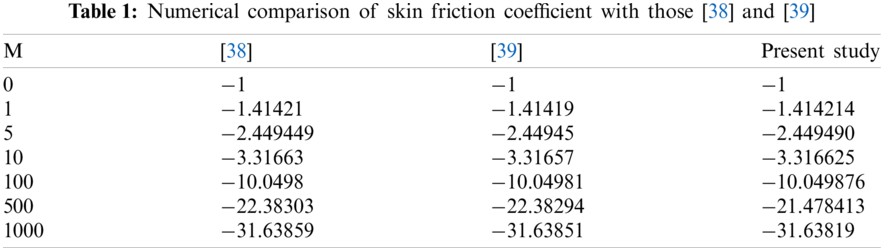5  Result and Discussion

5.1 The Skin Friction, Nusselt Number, and Density Number

Table 2 is presented to study the impact of important parameters on skin friction CfxRe0.5. The skin friction coefficient CfxRe0.5 is declined as the thermal Grashof number Gr, the Rayleigh number Rb, the power-law index n, and the velocity power index m is boosted, whereas it increases as the magnetic parameter is enhanced. Table 3 is presented to view the variations in Nusselt number due to some important parameters. The heat transfer rate NuxRe0.5 is hiked as Brownian motion parameter Nb and the thermal radiation parameter Rd whereas NuxRe0.5 is declined as the velocity power index m, the Eckert number Ec and thermophoresis parameter Nt is hiked. From Table 4, it is noticeable that the density number NnxRe0.5 is enhanced as the bioconvection Peclet number Pe and bioconvection Rayleigh number Rb is upsurged, whereas it decreases for increased values of each velocity power index m, the Schmidt number for motile microorganism and strength of the homogeneous reaction K.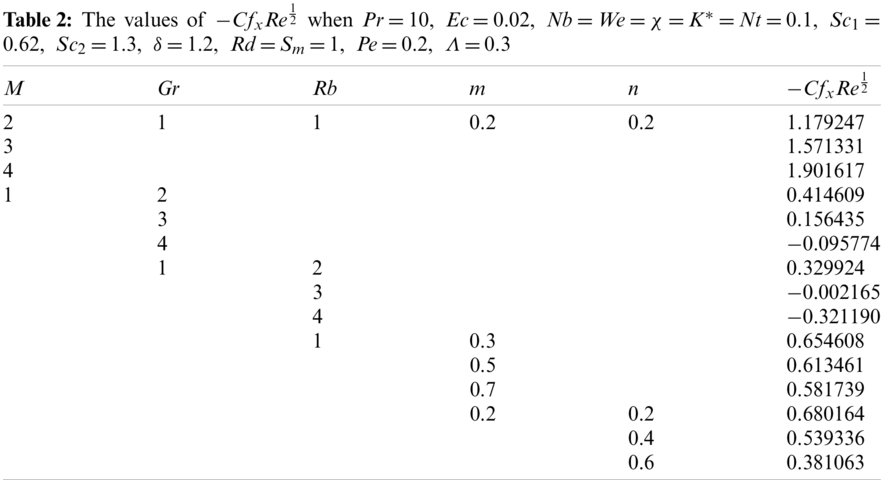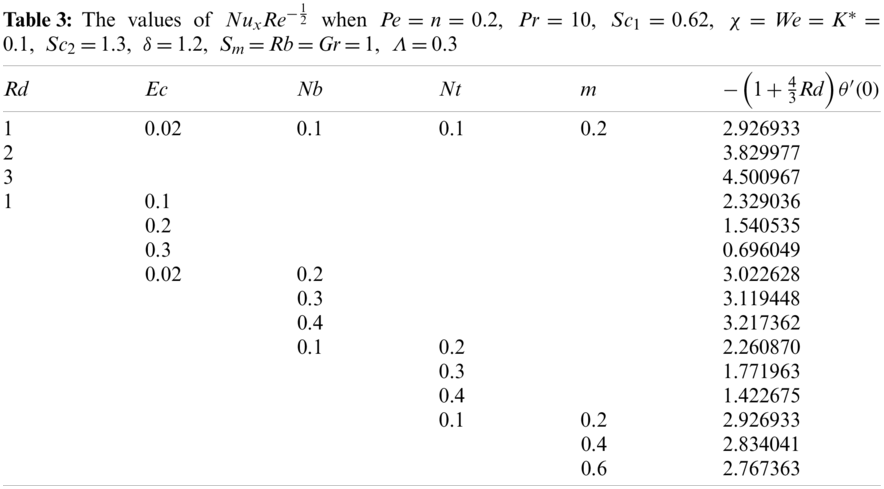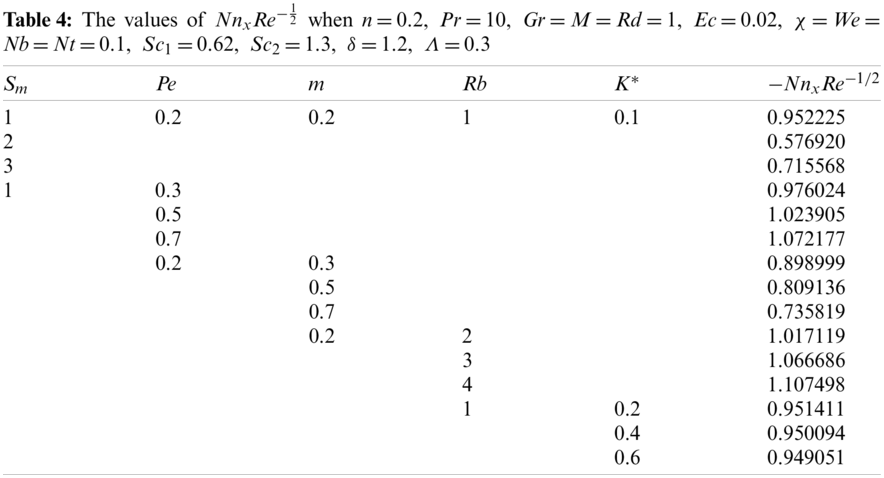5.2 Graphical Results

The impact of the sundry parameters on different profiles is presented graphically and discussed in detail. For the whole study, the values of the parameters are considered as m=0.25,Pe=n=0.2,Pr=10,Rb=Sm=Gr=M=Rd=1,Ec=0.02,χ=Nb=K=Nt=We=0.1,Sc1=0.62,Sc2=1.3,δ=1.2,Λ=0.3 and the varying parameter is displayed in the respective figure.

Figs. 2a2d are sketched to study the impact of the Brownian motion parameter Nb. An increment in Nb causes the tangent hyperbolic nanofluid to move up the horizontal surface. By the Brownian motion theory, the speed of nanoparticles is directly proportional to temperature. With the rises in temperature, the nanoparticles have more kinetic energy-yielding, movement faster. With an increment in Nb, the motion of the nanoparticles is enhanced, due to which the temperature is enhanced . The same result is obtained; however, due to replications, it is not presented. Due to that, enhancement of the temperature causes a hike in the concentration of the homogeneous bulk fluid X, and as a consequence, the concentration gradient of the bulk fluid H is declined, as presented in Figs. 2a2b. Fig. 2c shows that the concentration of heterogeneous fluid G declined as Nb is upsurged, whereas an overshoot is noticed in the gradient of the concentration of the reactant Y near ups 0<η0.8 approximately as shown in Fig. 2d. The variations due to thermophoresis parameter Nt on temperature θ(η), temperature gradient θ(η), Concentration of the bulk fluid (homogeneous) H(η) gradient of the concentration bulk fluid H(η), Concentration of the reactant Y (heterogeneous) G(η) and its gradient H(η) are divulged in Figs. 3a3f. An increment in Nt causes an increase in the temperature of the nanofluid, and the temperature gradient of the nanofluid is declined. Similarly, the concentration of the reactant X is diminished, and its gradient is hiked. Physically, in thermophoresis, the particles apply a force on the other particles, due to which the particles move towards the lower temperature region. Therefore, an increment in Nt means more application of the force on the other particles, due to which more fluid moves from the hotter region to the colder region. Consequently, the concentration of the bulk fluid X is reduced, and the concentration of the reactant Y (heterogeneous) is hiked. Inside the domain, H(η) is small whereas, G(η) is higher for the increasing values of Nt.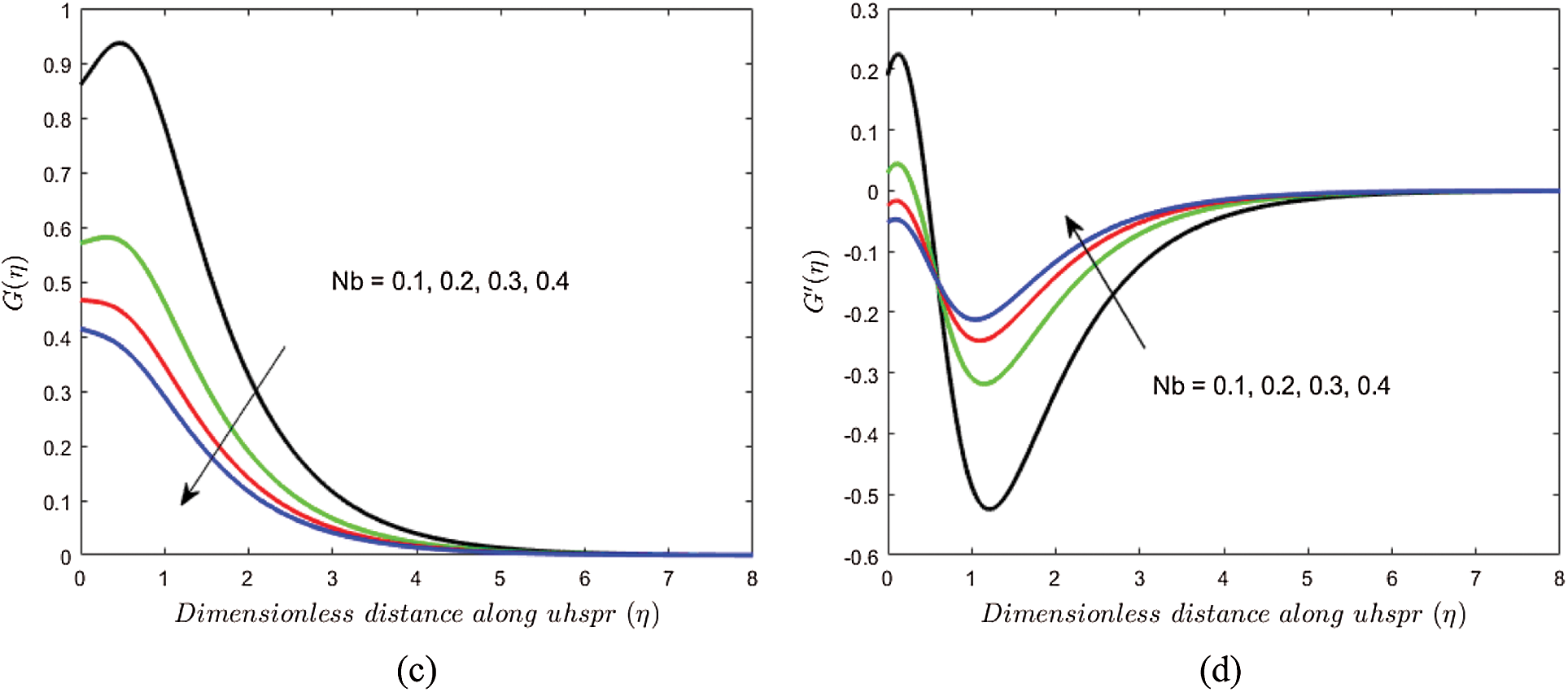Figure 2: Variations in (a) H(η), (b) H(η), (c) G(η) and (d) G(η) due to Nb

In order to view the influence of thermal Grashof number Gr on the horizontal velocity f(η) and motile density profile L(η) are displayed in Figs. 4a4b. From these graphs, it is evident that a boost in Gr results in a hike in the horizontal velocity of ups, whereas the diffusion of the motile microorganism is declined. Physically, the buoyancy force of the flow field is enhanced as the Grashof number Gr is hiked. Moreover, the increasing values of Gr have a negligible impact on L(η) near ups. The fluctuation due to bioconvection Rayleigh number Rb on horizontal velocity f(η) and motile density profile L(η) are presented in Figs. 5a5b. From the graphs of these figures, it is noticed that f(η) is hiked, and this increase is more significant near the surface as Rb is boosted. Whereas the diffusion of the motile microorganism is depreciated. The fluctuation due to the magnetic parameter M on f(η) and L(η) is presented in Figs. 6a6b. Increasing values of M enhance the resistive force, due to which the f(η) is declined, whereas the tendency of swimming off the self-propelled microorganisms near the boundary layer increased due to which the microorganism flux grows, as shown in Fig. 6b. This verifies the general behavior of the magnetic effect.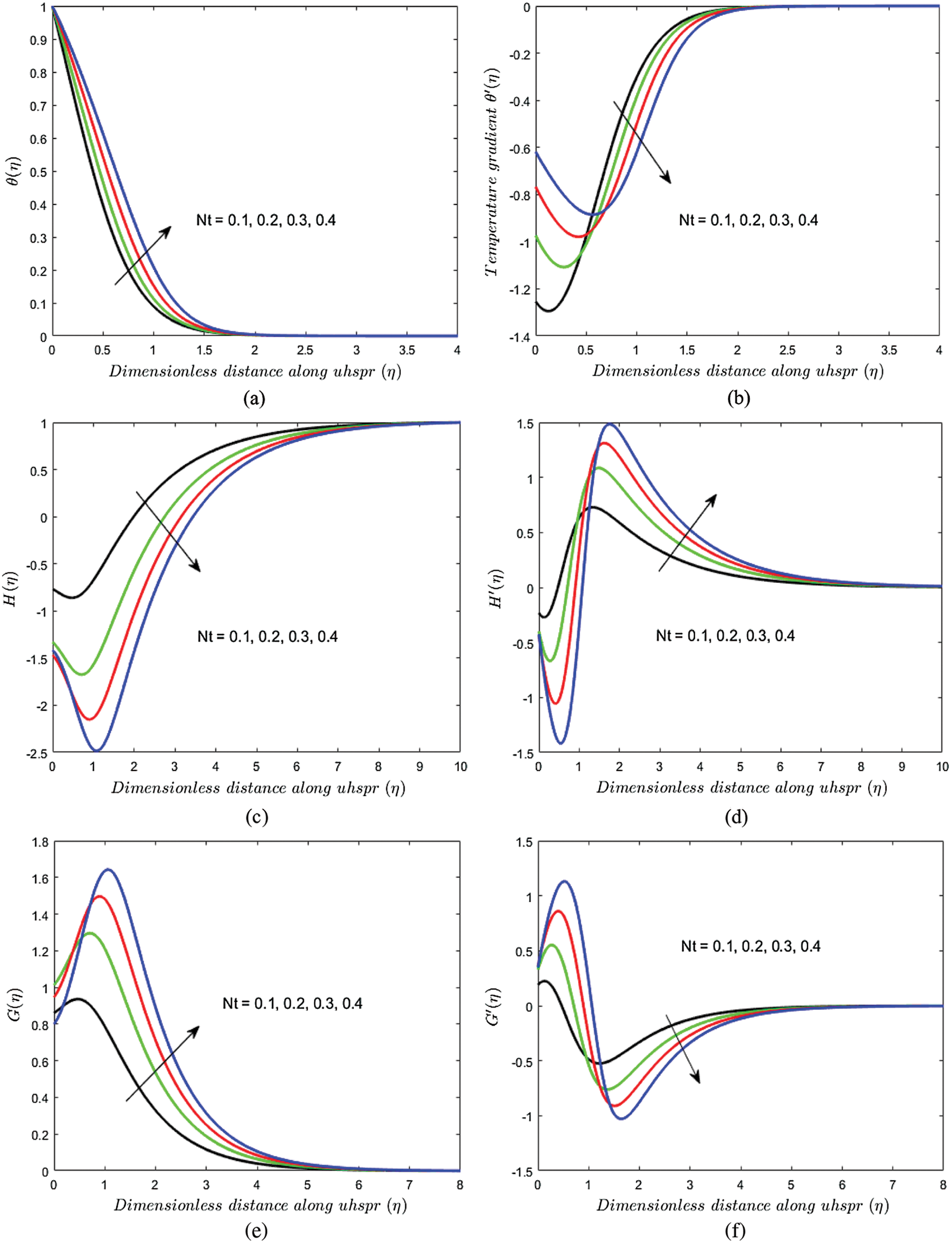Figure 3: Variations in (a) θ(η) (b) θ(η), (c) H(η), (d) H(η), (e) G(η) and (f) G(η) due to Nt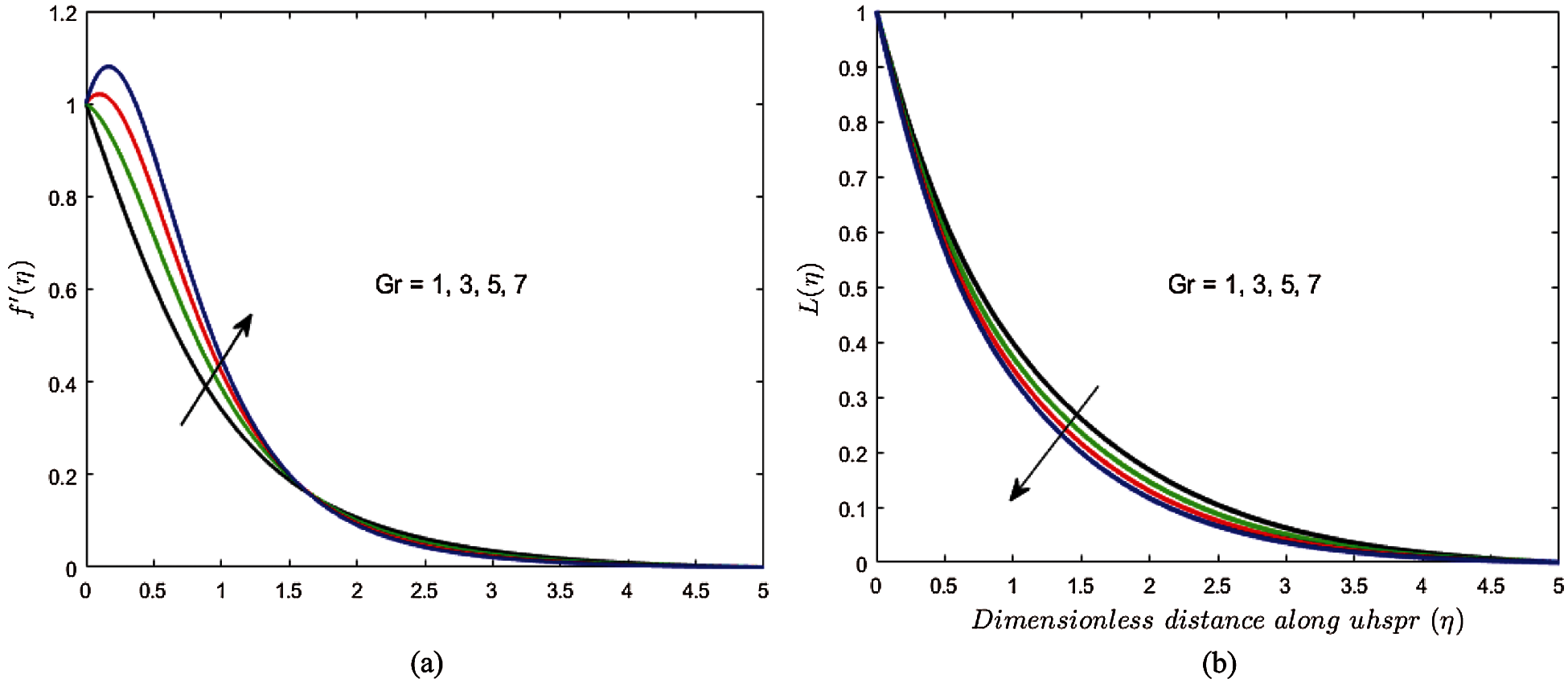Figure 4: Variations in (a) f(η) and (b) L(η) due to GrFigure 5: Variations in (a) f(η) and (b) L(η) due to Rb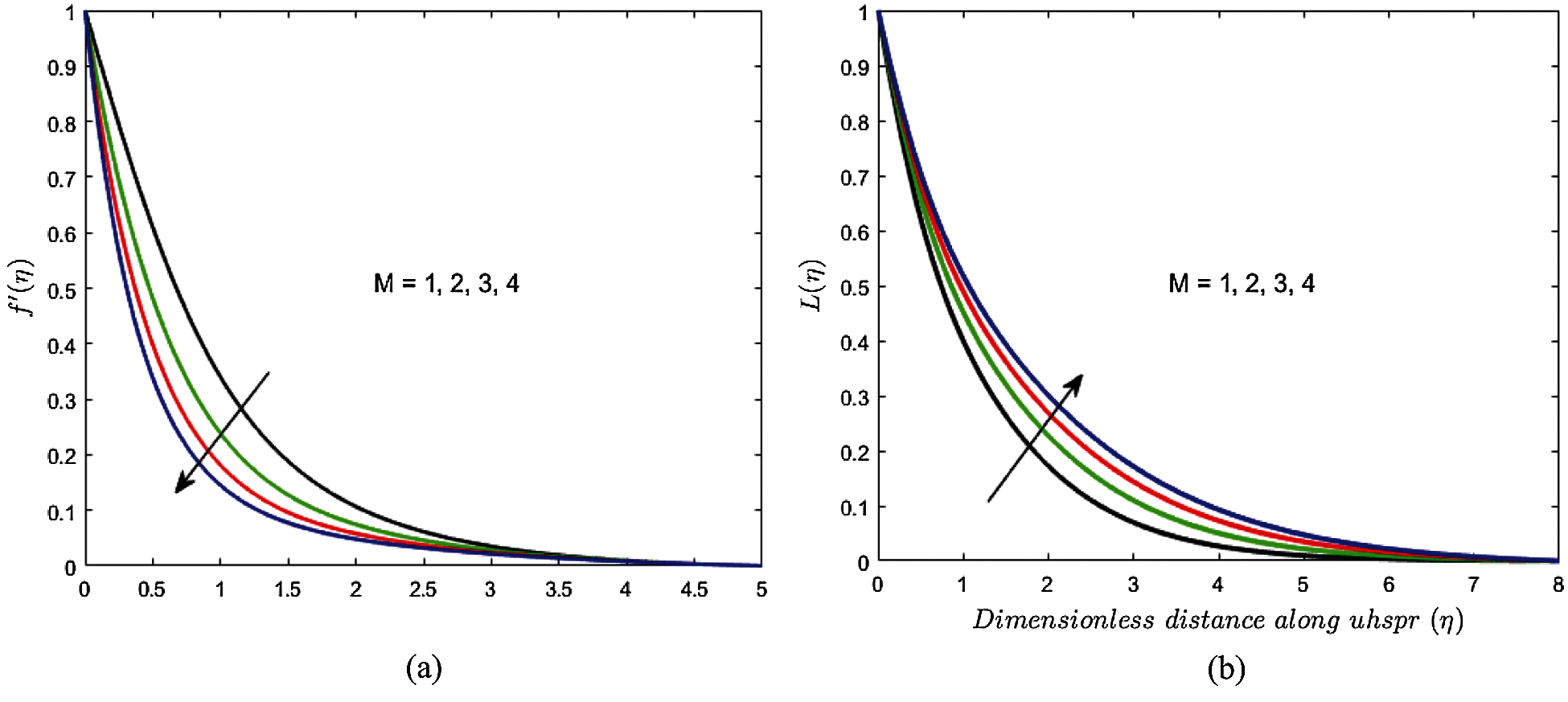Figure 6: Variations in (a) f(η) and (b) L(η) due to M

6  Conclusions

In this numerical study, the tangent hyperbolic nanofluid past the upper horizontal surface of paraboloid revolution with quartic autocatalysis chemical reaction has been studied. A few of the key results are:

•   The velocity profile is declined, whereas the density profile is enhanced as the magnetic parameter is boosted.

•   The impact of the Brownian motion parameter on the homogeneous fluid is increasing, whereas the thermophoresis parameter has the opposite impact.

•   The density profile of motile microorganisms is hiked as the bioconvection Rayleigh number is upsurged, whereas it decreases for the increasing values of the thermal Grashof number.

•   The Nusselt number and motile density number declined as the velocity power index was enhanced.

•   The Skin friction coefficient is declined as each of the thermal Grashof numbers, power-law index, velocity power index, and bioconvection Rayleigh number are boosted.

•   The influence of the Brownian motion parameter on the heterogeneous fluid is decreasing, whereas the opposite trend is noticed for the thermophoresis parameter.

Acknowledgement: We thank Dr. Muhammad Kashif Iqbal for his assistance in proofreading the manuscript.

Author's Contributions: All authors equally contributed to this work. All authors read and approved the final manuscript.

Funding Statement: The authors received no external funding for this study.

Conflicts of Interest: The authors declare that they have no conflicts of interest to report regarding the present study.

References

1.  1.  Hassan, M., Ellahi, R., Bhatti, M. M., Zeeshan, A. (2019). A comparative study on magnetic and non-magnetic particles in nanofluid propagating over a wedge. Canadian Journal of Physics, 97(3), 277–285. DOI 10.1139/cjp-2018-0159.
2.  2.  Raza, J. (2019). Thermal radiation and slip effects on magnetohydrodynamic (MHD) stagnation point flow of casson fluid over a convective stretching sheet. Propulsion and Power Research, 8(2), 138–146. DOI 10.1016/j.jppr.2019.01.004.
3.  3.  Yashkun, U., Zaimi, K., Bakar, N. A. A., Ishak, A., Pop, I. (2020). MHD hybrid nanofluid flow over a permeable stretching/shrinking sheet with thermal radiation effect. International Journal of Numerical Methods for Heat & Fluid Flow, 31(3), 1014–1031. DOI 10.1108/HFF-02-2020-0083.
4.  4.  Anuar, N. S., Bachok, N., Turkyilmazoglu, M., Arifin, N. M., Rosali, H. (2020). Analytical and stability analysis of MHD flow past a nonlinearly deforming vertical surface in carbon nanotubes. Alexandria Engineering Journal, 59(1), 497–507. DOI 10.1016/j.aej.2020.01.024.
5.  5.  Kamran, A., Hussain, S., Sagheer, M., Akmal, N. (2017). A numerical study of magnetohydrodynamics flow in casson nanofluid combined with joule heating and slip boundary conditions. Results in Physics, 7, 3037–3048. DOI 10.1016/j.rinp.2017.08.004.
6.  6.  Sajid, M., Shahzad, H., Mughees, M., Ali, N. (2019). Mathematical modeling of slip and magnetohydrodynamics effects in blade coating. Journal of Plastic Film & Sheeting, 35(1), 9–21. DOI 10.1177/8756087918777782.
7.  7.  Lund, L. A., Omar, Z., Khan, I., Raza, J., Sherif, E. S. M. et al. (2020). Magnetohydrodynamic (MHD) flow of micropolar fluid with effects of viscous dissipation and joule heating over an exponential shrinking sheet: Triple solutions and stability analysis. Symmetry, 12(1), 142. DOI 10.3390/sym12010142.
8.  8.  Atif, S. M., Hussain, S., Sagheer, M. (2018). Numerical study of MHD micropolar carreau nanofluid in the presence of an induced magnetic field. AIP Advances, 8(3), 035219. DOI 10.1063/1.5022681.
9.  9.  Atif, S. M., Kamran, A., Shah, S. (2021). MHD micropolar nanofluid with non-Fourier and non-fick's law. International Communications in Heat and Mass Transfer, 122, 105114. DOI 10.1016/j.icheatmasstransfer.2021.105114.
10. 10. Khan, A., Gul, T., Zaheer, Z., Amiri, I. S. (2019). The flow of ferromagnetic nanofluid over an extending surface under the effect of operative prandtl model: A numerical study. Advances in Mechanical Engineering, 11(12), DOI 10.1177/1687814019896128.
11. 11. Atif, S. M., Hussain, S., Sagheer, M. (2019). Effect of thermal radiation on MHD micropolar Carreau nanofluid with viscous dissipation. Joule Heating, and Internal Heating. Scientia Iranica, 26(6), 3875–3888. DOI 10.24200/SCI.2019.51653.2294.
12. 12. Ahmed, A., Khan, M., Hafeez, A., Ahmed, J. (2020). Thermal analysis in unsteady radiative Maxwell nanofluid flow subject to heat source/sink. Applied Nanoscience, 10, 5489–5497. DOI 10.1007/s13204-020-01431-w.
13. 13. Tlili, I., Shahmir, N., Ramzan, M., Kadry, S., Kim, J. Y. et al. (2020). A novel model to analyze darcy forchheimer nanofluid flow in a permeable medium with entropy generation analysis. Journal of Taibah University for Science, 14(1), 916–930. DOI 10.1080/16583655.2020.1790171.
14. 14. Khan, M., Rasheed, A., Salahuddin, T. (2020). Radiation and chemical reactive impact on tangent hyperbolic fluid flow having double stratification. AIP Advances, 10(7), 075211. DOI 10.1063/5.0003717.
15. 15. Ahmad, R., Farooqi, A., Zhang, J., Ali, N. (2019). Steady flow of a power law fluid through a tapered non-symmetric stenotic tube. Applied Mathematics and Nonlinear Sciences, 4(1), 255–266. DOI 10.2478/AMNS.2019.1.00022.
16. 16. Ghasemi, S., Hatami, M. (2021). Solar radiation effects on MHD stagnation point flow and heat transfer of a nanofluid over a stretching sheet. Case Studies in Thermal Engineering, 25, 100898, DOI 10.1016/j.csite.2021.100898.
17. 17. Reddy, G. J., Kethireddy, B., Kumar, M., Hoque, M. M. (2018). A molecular dynamics study on transient non-newtonian MHD casson fluid flow dispersion over a radiative vertical cylinder with entropy heat generation. Journal of Molecular Liquids, 252, 245–262. DOI 10.1016/j.molliq.2017.12.077.
18. 18. Salawu, S. O., Ogunseye, H. A. (2020). Entropy generation of a radiative hydromagnetic powell-eyring chemical reaction nanofluid with variable conductivity and electric field loading. Results in Engineering, 5, 100072. DOI 10.1016/j.rineng.2019.100072.
19. 19. Hamid, A. (2020). Terrific effects of ohmic-viscous dissipation on casson nanofluid flow over a vertical thin needle: Buoyancy assisting & opposing flow. Journal of Materials Research and Technology, 9(5), 11220–11230. DOI 10.1016/j.jmrt.2020.07.070.
20. 20. Shah, S., Atif, S. M., Kamran, A. (2021). Radiation and slip effects on MHD Maxwell nanofluid flow over an inclined surface with chemical reaction. Heat Transfer, 50(4), 4062–4085. DOI 10.1002/htj.22064.
21. 21. Kuznetsov, A. V., Nield, D. A. (2010). Natural convective boundary-layer flow of a nanofluid past a vertical plate. International Journal of Thermal Sciences, 49(2), 243–247. DOI 10.1016/j.ijthermalsci.2009.07.015.
22. 22. Kuznetsov, A. V., Nield, D. A. (2011). Double-diffusive natural convective boundary-layer flow of a nanofluid past a vertical plate. International Journal of Thermal Sciences, 50(5), 712–717. DOI 10.1016/j.ijthermalsci.2011.01.003.
23. 23. Nima, N. I., Salawu, S. O., Ferdows, M., Shamshuddin, M. D., Alsenafi, A. et al. (2020). Melting effect on non-newtonian fluid flow in gyrotactic microorganism saturated non-darcy porous media with variable fluid properties. Applied Nanoscience, 10(10), 3911–3924. DOI 10.1007/s13204-020-01491-y.
24. 24. Khan, S. U., Tlili, I. (2020). Significance of activation energy and effective prandtl number in accelerated flow of jeffrey nanoparticles with gyrotactic microorganisms. Journal of Energy Resources Technology, 142(11). DOI 10.1115/1.4047248.
25. 25. Kotha, G., Kolipaula, V. R., Rao, M. V. S., Penki, S., Chamkha, A. J. (2020). Internal heat generation on bioconvection of an MHD nanofluid flow due to gyrotactic microorganisms. The European Physical Journal Plus, 135(7), 1–19. DOI 10.1140/epjp/s13360-020-00606-2.
26. 26. Atif, S. M., Hussain, S., Sagheer, M. (2019). Magnetohydrodynamic stratified bioconvective flow of micropolar nanofluid due to gyrotactic microorganisms. AIP Advances, 9(2), 025208. DOI 10.1063/1.5085742.
27. 27. Animasaun, I. L. (2015). Effects of thermophoresis, variable viscosity and thermal conductivity on free convective heat and mass transfer of non-darcian MHD dissipative casson fluid flow with suction and nth order of chemical reaction. Journal of the Nigerian Mathematical Society, 34(1), 11–31. DOI 10.1016/j.jnnms.2014.10.008.
28. 28. Lotka, A. J. (2002). Contribution to the theory of periodic reactions. The Journal of Physical Chemistry, 14(3), 271–274. DOI 10.1021/j150111a004.
29. 29. Sapre, A. V. (1989). Diffusional enhancement of autocatalytic reactions in catalyst particles. AIChE Journal, 35(4), 655–657. DOI 10.1002/aic.690350416.
30. 30. Animasaun, I. L., Raju, C. S. K., Sandeep, N. (2016). Unequal diffusivities case of homogeneous–heterogeneous reactions within viscoelastic fluid flow in the presence of induced magnetic-field and nonlinear thermal radiation. Alexandria Engineering Journal, 55(2), 1595–1606. DOI 10.1016/j.aej.2016.01.018.
31. 31. Patil, P. M., Roy, S., Moitsheki, R. J., Momoniat, E. (2017). Double diffusive flows over a stretching sheet of variable thickness with or without surface mass transfer. Heat Transfer—Asian Research, 46(8), 1087–1103. DOI 10.1002/htj.21261.
32. 32. Qasim, M., Riaz, N., Lu, D., Afridi, M. I. (2020). Mixed convection flow over a stretching sheet of variable thickness: Analytical and numerical solutions of self-similar equations. Heat Transfer, 49(6), 3882–3899. DOI 10.1002/htj.21813.
33. 33. Abdeljawad, T., Ullah, A., Alrabaiah, H., Ullah, I., Ayaz, M. et al. (2020). Thermal radiations and mass transfer analysis of the three-dimensional magnetite carreau fluid flow past a horizontal surface of paraboloid of revolution. Processes, 8(6), 656. DOI 10.3390/pr8060656.
34. 34. Khan, M., Hussain, A., Malik, M. Y., Salahuddin, T. (2017). Biconvection flow of carreau fluid over an upper paraboloid surface: A computational study. Results in Physics, 7, 4050–4057. DOI 10.1016/j.rinp.2017.10.023.
35. 35. Santoshi, P. N., Reddy, G. V. R., Padma, P. (2020). Flow features of non-newtonian fluid through a paraboloid of revolution. International Journal of Applied and Computational Mathematics, 6, 1–22. DOI 10.1007/s40819-020-00828-z.
36. 36. Chaudhary, M. A., Merkin, J. H. (1995). A simple isothermal model for homogeneous-heterogeneous reactions in boundary-layer flow. I Equal diffusivities. Fluid Dynamics Research, 16(6), 311–333. DOI 10.1016/0169-5983(95)00015-6.
37. 37. Lynch, D. T. (1992). Chaotic behavior of reaction systems: Mixed cubic and quadratic autocatalysis. Chemical Engineering Science, 47(17–18), 4435–4444. DOI 10.1016/0009-2509(92)85121-Q.
38. 38. Akbar, N. S., Ebaid, A., Khan, Z. H. (2015). Numerical analysis of magnetic field effects on eyring-powell fluid flow towards a stretching sheet. Journal of Magnetism and Magnetic Materials, 382, 355–358. DOI 10.1016/j.jmmm.2015.01.088.
39. 39. Malik, M. Y., Salahuddin, T., Hussain, A., Bilal, S. (2015). MHD flow of tangent hyperbolic fluid over a stretching cylinder: Using keller box method. Journal of Magnetism and Magnetic Materials, 395, 271–276. DOI 10.1016/j.jmmm.2015.07.097.
40. 40. Zaimi, K., Ishak, A., Pop, I. (2014). Boundary layer flow and heat transfer over a nonlinearly permeable stretching/shrinking sheet in a nanofluid. Scientific Reports, 4(1), 1–8. DOI 10.1038/srep04404.This work is licensed under a Creative Commons Attribution 4.0 International License, which permits unrestricted use, distribution, and reproduction in any medium, provided the original work is properly cited.